## 8.1 Overview

Outcome Predictor Normality? Linearity? Analysis Method
Interval Binary Yes 2-sample $$t$$-test or linear regression
Ordinal Binary No Wilcoxon 2-sample test or proportional odds model
Categorical Categorical Pearson $$\chi^2$$ test
Interval Interval Yes Yes Correlation or linear regression
Ordinal Ordinal No No Spearman’s rank correlation
• Examine association between continuous/interval outcome ($$y$$) and continuous/interval predictor ($$x$$)
• Scatterplot of $$y$$ versus $$x$$
• Nonparametric smoother, including local linear regression, “super smoother”, and overlapping moving windows for any statistic of interest

## 8.2 Pearson’s correlation coefficient

• $$r = \frac{\Sigma(x_i - \bar{x})(y_i - \bar{y})}{\sqrt{\Sigma(x_i - \bar{x})^2\Sigma(y_i - \bar{y})^2}}$$
• Range: $$-1 \leq r \leq 1$$
• Correlation coefficient is a unitless index of strength of association between two variables (+ = positive association, - = negative, 0 = no association)
• Measures the linear relationship between $$X$$ and $$Y$$
• Can test for significant association by testing whether the population correlation is zero

$t = \frac{r\sqrt{n-2}}{\sqrt{1-r^{2}}}$

which is identical to the $$t$$-test used to test whether the population $$r$$ is zero; d.f.=$$n-2$$.

• Use probability calculator for $$t$$ distribution to get $$P$$-value (2-tailed if interested in association in either direction)
• 1-tailed test for a positive correlation between $$X$$ and $$Y$$ tests $$H_{0}:$$ when $$X \uparrow$$ does $$Y \uparrow$$ in the population?
• Confidence intervals for population $$r$$ calculated using Fisher’s $$Z$$ transformation

$Z = \frac{1}{2} \mathrm{log}_\mathrm{e} \left( \frac{1+r}{1-r} \right)$

• For large $$n$$, Z follows a Normal distribution with standard error $$\frac{1}{\sqrt{n-3}}$$
• To calculate a confidence interval for $$r$$, first find the confidence interval for $$Z$$ then transform back to the $$r$$ scale $\begin{array}{ccc} Z & = & \frac{1}{2} \mathrm{log}_\mathrm{e} \left( \frac{1+r}{1-r} \right) \\ 2*Z & = & \mathrm{log}_\mathrm{e} \left( \frac{1+r}{1-r} \right) \\ \mathrm{exp}(2Z) & = & \left( \frac{1+r}{1-r} \right) \\ \mathrm{exp}(2Z) * (1-r) & = & 1 + r \\ \mathrm{exp}(2Z) - r * \mathrm{exp}(2Z) & = & 1 + r \\ \mathrm{exp}(2Z) - 1 & = & r * \mathrm{exp}(2Z) + r \\ \mathrm{exp}(2Z) - 1 & = & r \left(\mathrm{exp}(2Z) + 1\right) \\ \frac{\mathrm{exp}(2Z) - 1}{\mathrm{exp}(2Z) + 1} & = & r \\ \end{array}$
• Example (Altman 89-90): Pearson’s $$r$$ for a study investigating the association of basal metabolic rate with total energy expenditure was calculated to be $$0.7283$$ in a study of $$13$$ women. Derive a 0.95 confidence interval for $$r$$.

$Z = \frac{1}{2} \mathrm{log}_\mathrm{e} \left( \frac{1+0.7283}{1-0.7283} \right) = 0.9251$

The lower limit of a 0.95 CI for $$Z$$ is given by

$0.9251 - 1.96 \times \frac{1}{\sqrt{13-3}} = 0.3053$

and the upper limit is

$0.9251 + 1.96 \times \frac{1}{\sqrt{13-3}} = 1.545$

A 0.95 CI for the population correlation coefficient is given by transforming these limits from the $$Z$$ scale back to the $$r$$ scale

$\frac{\mathrm{exp}(2\times 0.3053) - 1}{\mathrm{exp}(2\times 0.3053) + 1} ~~~ \mathrm{to} ~~~ \frac{\mathrm{exp}(2\times 1.545) - 1}{\mathrm{exp}(2\times 1.545) + 1}$

Which gives a 0.95 CI from 0.30 to 0.91 for the population correlation

Code
n <- 13
r <- 0.7283
z.transform <- 0.5 * log((1 + r) / (1 - r))
clz <- z.transform + c(-1, 1) * qnorm(0.975) / sqrt(n - 3)
clr <- (exp(2 * clz) - 1) / (exp(2 * clz) + 1)
round(c(z.transform, clz, clr), 4)
 0.9251 0.3053 1.5449 0.2962 0.9129

## 8.3 Spearman’s Rank Correlation

• Pearson’s $$r$$ assumes linear relationship between $$X$$ and $$Y$$
• Spearman’s $$\rho$$ (sometimes labeled $$r_{s}$$) assumes monotonic relationship between $$X$$ and $$Y$$
• when $$X \uparrow$$ $$Y$$ always $$\uparrow$$ or stays flat, or $$Y$$ always $$\downarrow$$ or stays flat
• does not assume linearity
• $$\rho = r$$ once replace column of $$X$$s by their ranks and column of $$Y$$s by ranks
• To test $$H_{0}:\rho=0$$ without assuming linearity or normality, being damaged by outliers, or sacrificing much power (even if data are normal), use a $$t$$ statistic:

$t = \frac{\rho\sqrt{n-2}}{\sqrt{1-\rho^{2}}}$

which is identical to the $$t$$-test used to test whether the population $$r$$ is zero; d.f.=$$n-2$$.

• Use probability calculator for $$t$$ distribution to get $$P$$-value (2-tailed if interested in association in either direction)
• 1-tailed test for a positive correlation between $$X$$ and $$Y$$ tests $$H_{0}:$$ when $$X \uparrow$$ does $$Y \uparrow$$ in the population?

## 8.4 Correlation Examples

• Correlation difficult to judge by eye
• Example plots on following pages
Code
# Generate 50 data points with Population correlations of 0, .2, .4, .6,
# .8, and .9 and plot results
require(ggplot2)
n <- 50
set.seed(123)
x <- rnorm(n, 5, 1)
d <- expand.grid(x=x, R=c(0, .2, .4, .6, .8, .9))
d <- transform(d, y = x + rnorm(nrow(d), 0,
ifelse(R == 0, 5, sqrt(R ^ -2 - 1))))
sfun <- function(i) {
x <- d$x[i]; y <- d$y[i]; R <- d$R[i] r <- cor(x, y) tr <- r * sqrt(n - 2) / sqrt(1 - r^2) rho <- cor(rank(x), rank(y)) trho <- rho * sqrt(n - 2) / sqrt(1 - rho^2) label <- paste('True r:', R, ' r:', round(r,2), ' t:', round(tr,2), ' rho:', round(rho,2), ' t:', round(trho,2), sep='') names(label) <- R label } stats <- tapply(1 : nrow(d), d$R, sfun)
d$stats <- factor(stats[as.character(d$R)], unique(stats))

ggplot(d, aes(x=x, y=y)) + geom_point() + facet_wrap(~ stats) +
theme(strip.text.x = element_text(size=7))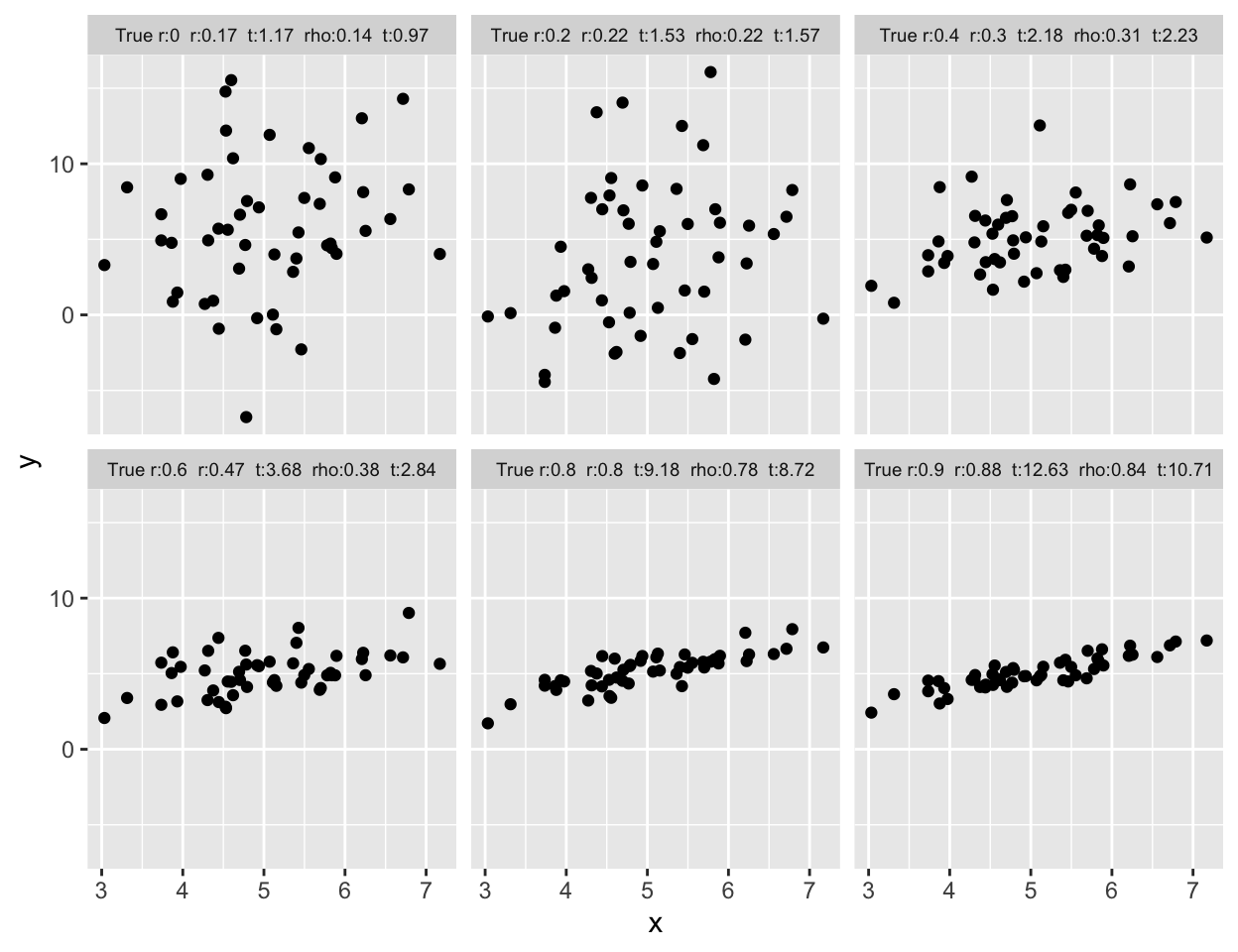Figure 8.1: Samples of size $$n=50$$ for X and Y are drawn from bivariate normal populations with true correlations ranging from 0.0 to 0.9. Pearson and Spearman sample correlations are shown for samples of size 50. Besides the population correlation coefficient, each panel is labeled with the estimated Pearson $$r$$, its $$t$$ statistic, the estimated Spearman $$\rho$$, and its $$t$$ statistic
Code
# Different scenarios that can lead to a correlation of 0.7
spar(mfrow=c(3,2))
set.seed(123)
rho <- 0.7; n <- 50
var.eps <- rho^-2 - 1
x <- rnorm(n, 5, 1)
y <- x + rnorm(n, 0, sqrt(var.eps))
cor(x,y)
 0.6951673
Code
plot(x,y,xlab='',ylab='')

x <- c(1:20,30)
y <- c(1:20,6.2)
cor(x,y)
 0.6988119
Code
plot(x,y,xlab='',ylab='')

set.seed(123)
x <- rnorm(40)
y <- rnorm(40)
x <- y <- 8.5
cor(x,y)
 0.7014825
Code
plot(x,y,xlab='',ylab='')

x <- rep(0:19,2)
y <- c(rep(.62,20),rep(2,20)) * x
cor(x,y)
 0.701783
Code
plot(x,y,xlab='',ylab='')

x <- -7:12
y <- x^2
cor(x,y)
 0.6974104
Code
plot(x,y,xlab='',ylab='')

set.seed(123)
tmp <- 1:20 / 2
x <- c(rnorm(20, tmp, 1), tmp + rnorm(20,14.5,1))
y <- c(rnorm(20, -tmp, 1), -tmp + rnorm(20,14.5,1))
cor(x,y)
 0.703308
Code
plot(x,y,xlab='',ylab='')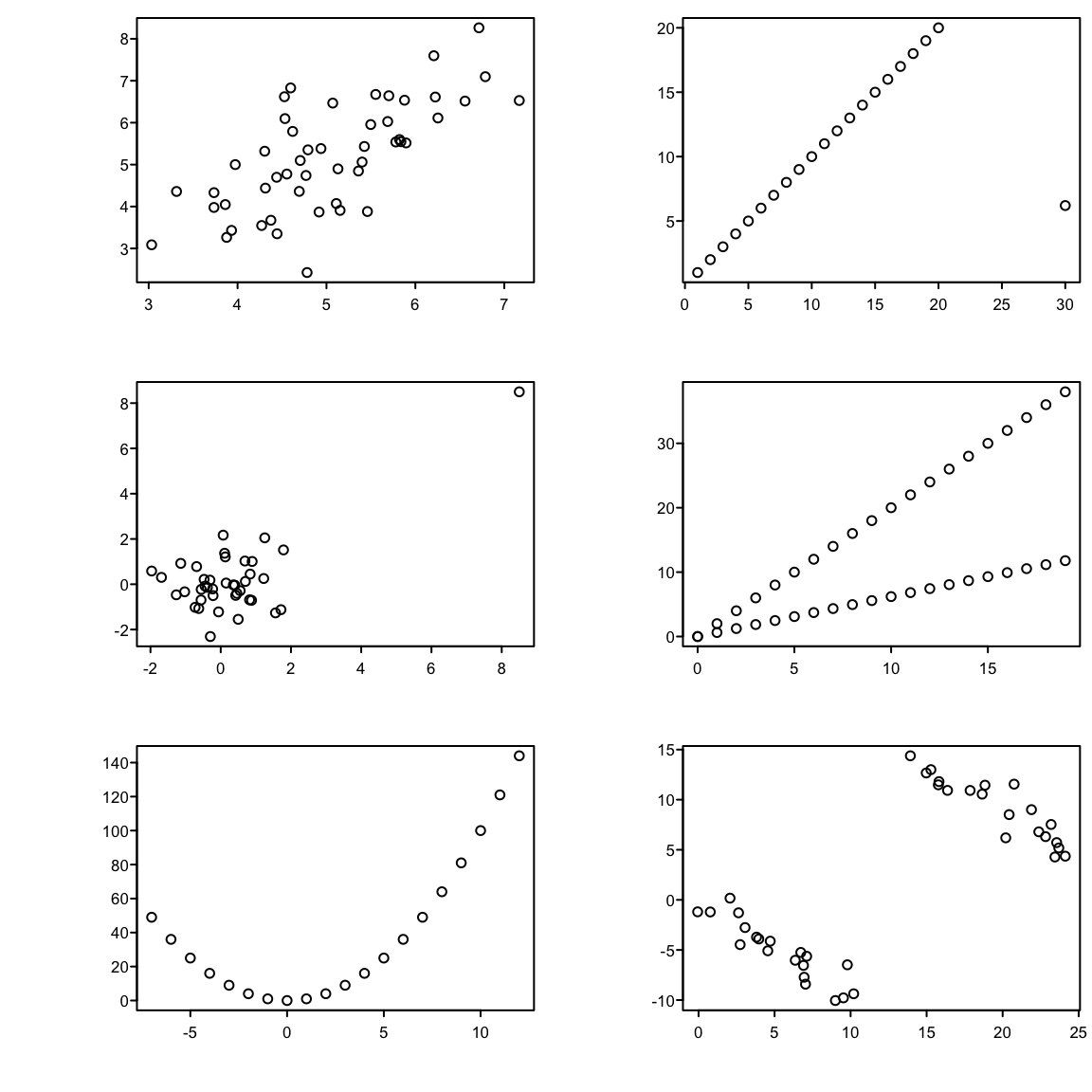Figure 8.2: Different observed datasets that have the same correlation. All six plots have a sample Pearson correlation of $$0.7$$.

## 8.5 Correlation and Agreement

• Compare two methods of measuring the same underlying value
• Lung function measured using a spirometer (expensive, accurate) or peak flow meter (cheap, less accurate)
• Two devices (oropharyngeal and conventional) used to measure acidity (pH) in the esophagus as a marker of reflux
• Typical (incorrect) approach begins with scatterplot of one method vs. the other with a 1:1 line indicating perfect agreement
• See Figure 4.11
• Incorrect approach would report a high correlation ($$r = 0.90$$) and conclude good agreement
• Problems with the correlation approach
1. $$r$$ measures the degree of linear association between two variables, not the agreement. If, for example, the Sandhill consistently gave pH values that were 0.5 unit higher than the Restech, we could still have high correlation, but poor agreement between the two devices. We can have high correlation if the two devices lie closely to any line, not just a 1:1 line that indicates perfect agreement.
2. A change in scale does not affect correlation, but does influence agreement. For example, if the Sandhill always registered 2 times larger than the Restech, we would have perfect correlation but the agreement would get progressively worse for larger values of pH.
3. Correlation depends on the range of the data so that larger ranges lead to larger correlations. This can lead to vary strange interpretations
Pearson ($$r$$) and Spearman ($$\rho$$) correlations for Restech and Sandhill pH data. The correlation calculated using all of the data is larger than the correlation calculated using a restricted range of the data. However, it would be difficult to claim that the overall agreement is better than both the agreement when pH is less than 4 and when pH is greater than 4.
$$r$$ $$\rho$$
all data 0.90 0.73
avg pH $$\leq 4$$ 0.51 0.58
avg pH $$> 4$$ 0.74 0.65
1. Tests of significance (testing if $$r=0$$) are irrelevant to the question at hand, but often reported to demonstrate a significant association. The two devices are measuring the same quantity, so it would be shocking if we did not observe a highly significant $$p$$-value. A $$p < .0001$$ is not impressive. A regression analysis with a highly significant slope would be similarly unimpressive.
2. Data can have high correlation, but poor agreement. There are many examples in the literature, but even in our analysis with $$r = 0.90$$, the correlation is high, but we will show that the agreement is not as good as the high correlation implies.

See Chapter 16 for simple approaches to assessing agreement and analyzing observer variability studies.

### 8.5.1 Bland-Altman Plots

• See Bland and Altman (1986, Lancet)
• Earlier: Tukey mean-difference plot
• Create plots of the difference in measurements on the y-axis versus the average value of the two devices on the x-axis
• If the two devices agree, the difference should be about zero
• The average of the two devices is our best estimate of the true, unknown (pH) value that is we are trying to measure
• Measurements will often vary in a systematic way over the range of measurement. By plotting the difference versus the average, we can visually determine if the difference changes over our estimate of the truth.
• Solid line indicated the mean, dashed lines are approximate 0.95 confidence intervals (assuming Normality)

But there is controversy about what should be on the $$x$$-axis of the plot. Krouwer Krouwer (2008) concluded that:

• When the two measures have nearly equal variability, i.e., when comparing two “field measurements”, the Bland-Altman approach is preferred
• When one measurement is a “reference standard” having much less variation than the field measurement, the reference standard and not the average of the two measurements should be on the $$x$$-axis
Code
require(Hmisc)
getHdata(esopH)
esopH$diff <- with(esopH, orophar - conv) ggplot(esopH, aes(x=(conv + orophar)/2, y=diff)) + stat_binhex(aes(alpha=..count.., color=Hmisc::cut2(..count.., g=20)), bins=80) + stat_smooth() + geom_hline(yintercept = mean(esopH$diff, na.rm=TRUE) +
c(-1.96, 0, 1.96) * sd(esopH$diff, na.rm=TRUE), linetype=c(2,1,2), color='brown') + xlab('Average of Conventional and Oropharyngeal pH') + ylab('Oropharyngeal Minus Conventional pH') + guides(alpha=FALSE, fill=FALSE, color=guide_legend(title='Frequency'))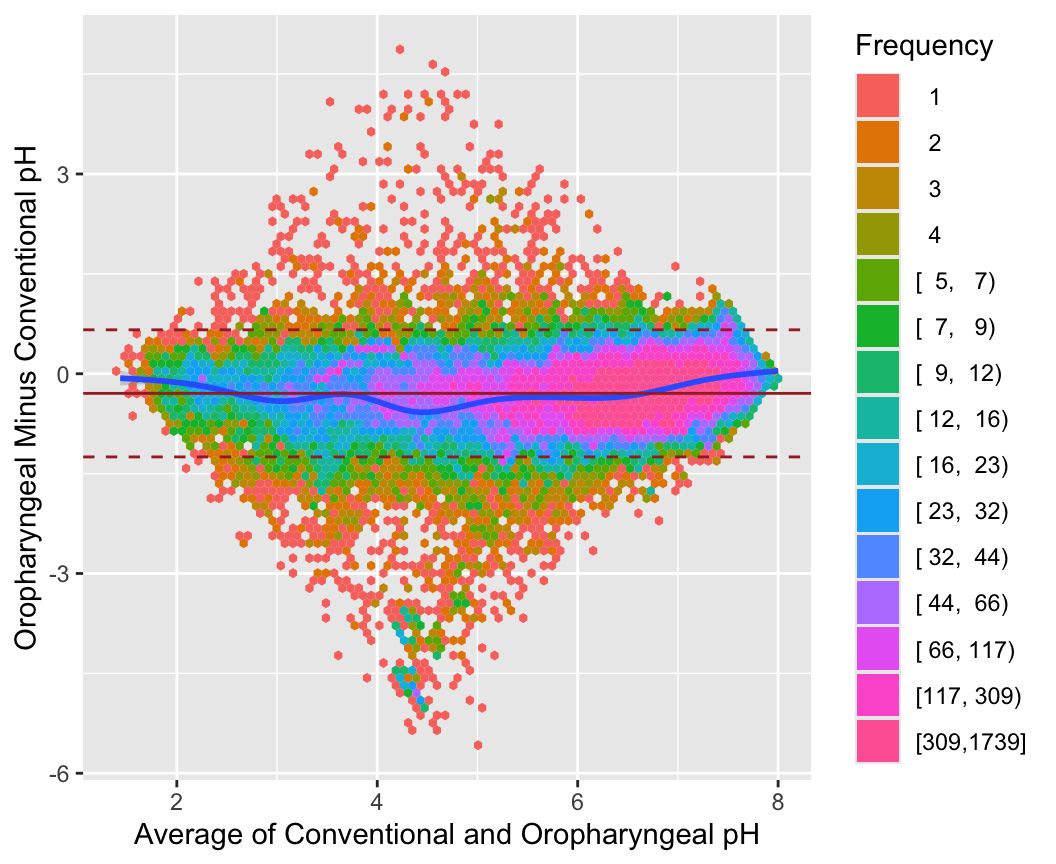Figure 8.3: Bland-Altman plot for the oroesophageal and conventional pH measurements, using hexagonal binning because of the large sample size. The difference in pH mesaurements (oro. -conventional) is presented on the $$y$$-axis and the average of the two devices on the $$x$$-axis. We see poor agreement around pH values of 4-5 • We will also consider differences in the two measurements over the time of day • The added smooth curve is called a locally weighted scatterplot smooth (loess) Code getHdata(esopH2) ggplot(esopH2, aes(x=time, y=diffpH)) + geom_point(pch='.') + stat_smooth() + geom_hline(yintercept = 0, col='gray60') + scale_x_continuous(breaks=seq(16, 38, by=4), labels=c("4 PM", "8 PM", "12 AM", "4 AM", "8AM", "12 PM"), limits=c(14, 14+24)) + ylab('Average of Oropharyngeal Minus Conventional pH') + xlab('Time of Day')Figure 8.4: Difference in pH measurements (oro. - conventional) by time of day along with a loess smoother and pointwise 0.95 confidence bands. Is the difference modified by a subject being in a supine position rather than being upright? ### 8.5.2 Sample Size for $$r$$ • Without knowledge of population variances, etc., $$r$$ can be useful for planning studies • Choose $$n$$ so that margin for error (half-width of C.L.) for $$r$$ is acceptable • Precision of $$r$$ in estimating $$\rho$$ is generally worst when $$\rho=0$$ • This margin for error as well as that for three other choices of the unknown true $$\rho$$ are shown in Figure 8.5. Code require(Hmisc) plotCorrPrecision(rho=c(0, .25, .5, .75), n=seq(10, 1000, length=100), ylim=c(0, .4), col=1:4, opts=list(keys='lines')) abline(h=seq(0, .4, by=0.025), v=seq(25, 975, by=25), col=gray(.9))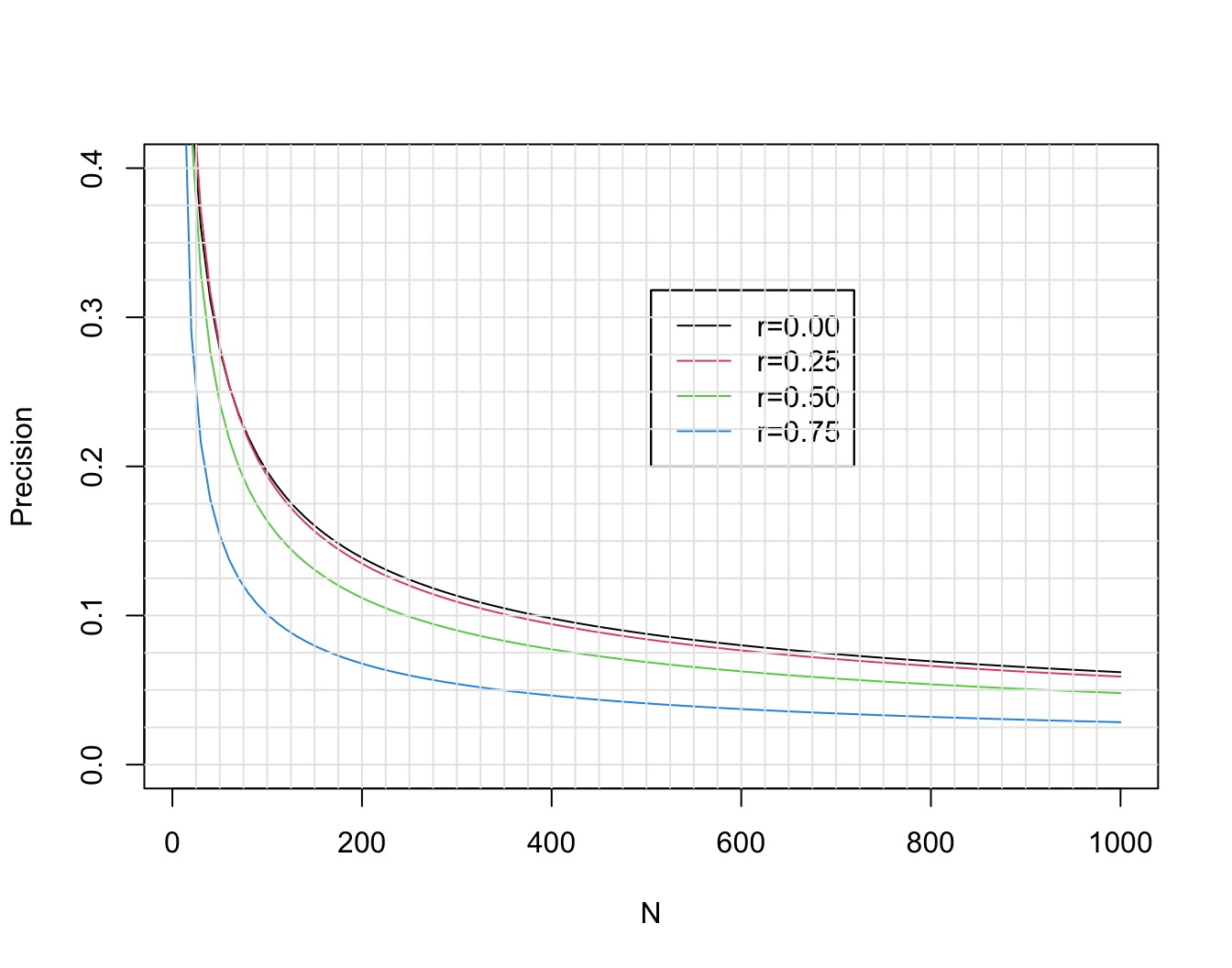Figure 8.5: Margin for error (length of longer side of asymmetric 0.95 confidence interval) for $$r$$ in estimating $$\rho$$, when $$\rho=0, 0.25, 0.5, 0.75$$. Calculations are based on Fisher $$z$$ transformation of $$r$$. ### 8.5.3 Comparing Two $$r$$’s • Rarely appropriate • Two $$r$$’s can be the same even though slopes may differ • Usually better to compare effects on a real scale (slopes) ## 8.6 Avoiding Overinterpretation • Often researchers • compute many correlations then • make a big deal out of the largest observed correlation • This is double dipping: using the same dataset to tell you which features to test, then testing those features • This is a ranking and selection problem, and the data seldom contain enough information to be reliable in the choices ### 8.6.1 Simulate Data • For our analysis experiments, simulate a sample of size 50 from a 10-variate normal distribution with a known correlation matrix • To specify this correlation matrix take the easy way out: compute an observed correlation matrix from a small sample where all correlations in the population are zero • The usual sample noise will generate some large observed correlations Code require(Hmisc) require(mvtnorm) # Get a population correlation matrix by getting sample correlations # on a random normal sample with N=20 and all true correlations=0 # then pretend these sample correlations were real population values set.seed(3) x <- rmvnorm(20, sigma=diag(10)) R <- rcorr(x)$r
# True correlations we will simulate from:
round(R, 2)
       [,1]  [,2]  [,3]  [,4]  [,5]  [,6]  [,7]  [,8]  [,9] [,10]
[1,]  1.00  0.01  0.47 -0.09 -0.31 -0.07  0.14  0.05  0.02 -0.49
[2,]  0.01  1.00  0.48  0.27  0.27  0.14  0.40 -0.17 -0.59  0.60
[3,]  0.47  0.48  1.00 -0.11  0.26 -0.31  0.45 -0.12 -0.50 -0.06
[4,] -0.09  0.27 -0.11  1.00  0.42  0.42 -0.07 -0.35  0.16  0.35
[5,] -0.31  0.27  0.26  0.42  1.00 -0.04  0.03 -0.40 -0.25  0.34
[6,] -0.07  0.14 -0.31  0.42 -0.04  1.00  0.19 -0.36  0.08  0.40
[7,]  0.14  0.40  0.45 -0.07  0.03  0.19  1.00 -0.37 -0.65 -0.07
[8,]  0.05 -0.17 -0.12 -0.35 -0.40 -0.36 -0.37  1.00  0.17 -0.24
[9,]  0.02 -0.59 -0.50  0.16 -0.25  0.08 -0.65  0.17  1.00 -0.18
[10,] -0.49  0.60 -0.06  0.35  0.34  0.40 -0.07 -0.24 -0.18  1.00
Code
# Get a huge sample from a multivariate normal distribution to see
# that it mimics the real correlation matrix R
x <- rmvnorm(50000, sigma=R)
table(round(R - rcorr(x)$r, 2))  -0.01 0 0.01 14 76 10  Code # Now sample from the population to get our dataset with N=50 x <- rmvnorm(50, sigma=R) rorig <- rcorr(x)$r
round(rorig, 2)
       [,1]  [,2]  [,3]  [,4]  [,5]  [,6]  [,7]  [,8]  [,9] [,10]
[1,]  1.00 -0.01  0.50  0.11  0.00 -0.16  0.07 -0.04 -0.04 -0.43
[2,] -0.01  1.00  0.50  0.19  0.43  0.13  0.60 -0.26 -0.76  0.69
[3,]  0.50  0.50  1.00 -0.15  0.45 -0.41  0.52 -0.18 -0.58 -0.01
[4,]  0.11  0.19 -0.15  1.00  0.45  0.30 -0.13 -0.35  0.09  0.14
[5,]  0.00  0.43  0.45  0.45  1.00 -0.12  0.27 -0.53 -0.42  0.20
[6,] -0.16  0.13 -0.41  0.30 -0.12  1.00  0.06 -0.27  0.00  0.51
[7,]  0.07  0.60  0.52 -0.13  0.27  0.06  1.00 -0.57 -0.81  0.19
[8,] -0.04 -0.26 -0.18 -0.35 -0.53 -0.27 -0.57  1.00  0.37 -0.13
[9,] -0.04 -0.76 -0.58  0.09 -0.42  0.00 -0.81  0.37  1.00 -0.41
[10,] -0.43  0.69 -0.01  0.14  0.20  0.51  0.19 -0.13 -0.41  1.00

### 8.6.2 Margin of Error for a Single $$r$$

• First compute the margin of error in estimating a single $$r$$ from $$n=50$$
• This is the spacing between $$r$$ and it’s lower 0.95 CL or the spacing between $$r$$ and its upper CL whichever is greatest
• CL based on Fisher’s $$z$$-transformation described earlier
• Compute this for 4 hypothetical true $$r$$: 0 0.25 0.5 0.75
Code
r <- (0 : 3) / 4
n <- 50
zcrit <- qnorm(0.975)
z <- 0.5 * log( (1 + r) / (1 - r))
lo <- z - zcrit/sqrt(n-3)
hi <- z + zcrit/sqrt(n-3)
rlo <- (exp(2*lo)-1)/(exp(2*lo)+1)
rhi <- (exp(2*hi)-1)/(exp(2*hi)+1)
w <- rbind(r=r, 'Margin of Error'=pmax(rhi - r, r - rlo))
prmatrix(round(w, 2), collab=rep('', 4))

r               0.00 0.25 0.50 0.75
Margin of Error 0.28 0.28 0.24 0.15
• If the true correlation is 0.5, the margin of error in estimating it is $$\pm 0.24$$ with $$n=50$$

### 8.6.3 Bootstrapping the Correlation Selection Process

• Can use the bootstrap to document the difficulty of the task
• Steps:
1. form a matrix with $$N$$ rows and $$p$$ columns where $$N$$ is the number of observations and $$p$$ is the number of variables being correlated with each other
2. draw a sample of size $$N$$ from this matrix by sampling its rows
3. compute all the correlation coefficients as was done previously
4. re-run the same selection process as was done on the original dataset
5. repeat 1000 times
6. examine the distribution of the selections over the 1000 repeats

### 8.6.4 Bootstrapping Bias in Largest Observed $$r$$

• Start with something simpler than ranking all the $$r$$s in the matrix: estimate the bias in the largest observed $$r$$
• Draw a sample with replacement from rows of the data matrix
• For each of these bootstrap samples find which pair of variables has the highest $$r$$
• Track this variable pair and compute $$r$$ in the original sample
• Compute the drop-off in $$r$$ from the bootstrap sample to the original sample
• This simulates the process used to find the largest $$r$$
• Example: use data simulated above with 10 standard normal random variables & known correlation matrix on 50 subjects
• Sample correlation matrix has $$\frac{10 \times 9}{2} = 45$$ distinct coefficients
Code
# Function to retrieve the upper triangle of a symmetric matrix
# ignoring the diagonal terms
up <- function(z) z[upper.tri(z)]
rorigu <- up(rorig)
max(rorigu)   # .685 = [2,10] element; 0.604 in population
 0.6854261
Code
which.max(rorigu)
 38
Code
# is the 38th element in the upper triangle

# Tabulate the difference between sample r estimates and true values
Ru <- up(R)
mean(abs(Ru - rorigu))
 0.1149512
Code
table(round(Ru - rorigu, 1))

-0.3 -0.2 -0.1    0  0.1  0.2
2    6    7    7   17    6 
Code
# Repeat the "finding max r" procedure for 1000 bootstrap samples
# Sample from x 1000 times with replacement, each time computing
# a new correlation matrix
samepair <- dropsum <- 0
for(i in 1 : 1000) {
b  <- sample(1 : 50, replace=TRUE)
xb <- x[b, ]   # sample with replacement from rows
r  <- rcorr(xb)$r ru <- up(r) wmax <- which.max(ru) if(wmax == 38) samepair <- samepair + 1 # Compute correlation for the bootstrap best pair in the original sample origr <- rorigu[wmax] # Compute drop-off in best r dropoff <- ru[wmax] - origr dropsum <- dropsum + dropoff } cat('Number of bootstaps selecting the original most correlated pair:', samepair, 'out of 1000', '\n') Number of bootstaps selecting the original most correlated pair: 642 out of 1000  Code cat('Mean dropoff for max r:', round(dropsum / 1000, 3), '\n') Mean dropoff for max r: 0.071  • For our dataset with $$n=50$$ we expect that the maximum observed $$r$$ out of 45 $$r$$s is biased high by 0.071 • Could subtract 0.071 to debias the observed max $$r$$ although this will worsen its precision ### 8.6.5 Bootstrapping Ranks of All $$r$$s • Do a more comprehensive assessment that quantifies the difficulty of the task in ranking all the $$r$$s • Quantity uncertainties in the ranks of the original correlation coefficients • Apparent “winner” was the one receiving the highest ranking $$q$$ among all $$r$$s • What is the distribution of $$q$$ over the 1000 bootstrap samples? • Can easily compute a 0.95 bootstrap nonparametric percentile confidence interval for the true unknown ranking of that feature combination and for ranking all the examined feature correlations • Bootstrap the correlation matrix and re-rank the coefficients • For each pair of variables compute the 0.95 confidence interval for the rank of its correlation from among the 45 Code # For each observed correlation compute its rank among 45 distinct pairs orig.ranks <- rank(up(rorig)) # Sample from x 1000 times with replacement, each time computing # a new correlation matrix Rm <- matrix(NA, nrow=1000, ncol=45) for(i in 1 : 1000) { b <- sample(1 : 50, replace=TRUE) xb <- x[b, ] r <- rcorr(xb)$r
Rm[i, ] <- rank(up(r))
}
# Over bootstrap correlations compute quantiles of ranks
low  <- apply(Rm, 2, quantile, probs=0.025)
high <- apply(Rm, 2, quantile, probs=0.975)
round(cbind('Original Rank'=orig.ranks, Lower=low, Upper=high))
      Original Rank Lower Upper
[1,]            22    12    34
[2,]            40    32    45
[3,]            41    30    45
[4,]            28    15    37
[5,]            31    20    38
[6,]            15     8    28
[7,]            24    11    34
[8,]            37    32    43
[9,]            38    32    43
[10,]            39    31    44
[11,]            14     8    27
[12,]            29    15    37
[13,]             9     3    15
[14,]            35    22    43
[15,]            18    10    26
[16,]            26    16    34
[17,]            44    38    45
[18,]            43    34    45
[19,]            16     9    27
[20,]            34    25    38
[21,]            25    14    34
[22,]            19    11    32
[23,]            12     7    21
[24,]            13     7    27
[25,]            10     4    20
[26,]             5     3    10
[27,]            11     5    22
[28,]             4     3     8
[29,]            20    10    33
[30,]             2     1     4
[31,]             3     2    11
[32,]            27    16    36
[33,]             7     4    13
[34,]            23    13    32
[35,]             1     1     2
[36,]            36    28    43
[37,]             6     3    15
[38,]            45    40    45
[39,]            21    12    31
[40,]            30    17    37
[41,]            33    21    39
[42,]            42    34    44
[43,]            32    20    38
[44,]            17    11    28
[45,]             8     3    16
• Highest observed $$r$$ (rank 45) has 0.95 CI $$[40,45]$$
• Data are consistent with it being the $$6^\textrm{th}$$ highest
• Smallest observed value (rank 1; most impressive negative correlation) has CI $$[1, 2]$$ for rank; data consistent with that pair of variables being the best or $$2^\textrm{nd}$$
• The $$r$$ originally ranked $$36^\textrm{th}$$ has a 0.95 CI of $$[28, 43]$$ so the data are consistent with it being in the top 3

### 8.6.6 Monte Carlo Simulation to Get A Rank Interval

• For comparison with the bootstrap, get the frequency distribution of ranks in repeated studies of the apparently highest $$r$$ in our $$n=50$$ study
• Repeated studies will also have $$n=50$$ and will be generated from the population correlation matrix
• Recall that original max $$r$$ was the $$38^\textrm{th}$$ element of the strung-out $$r$$ matrix from our sample
Code
ranks <- integer(1000)
for(i in 1 : 1000) {
xs <- rmvnorm(50, sigma=R)
rsim <- up(rcorr(xs)\$r)
ranks[i] <- rank(rsim)
}
table(ranks)   # freqs. of ranks of 38th element in new samples
ranks
34  35  36  37  38  39  40  41  42  43  44  45
2   1   6   8  10  18  25  38  58  88 152 594 
Code
quantile(ranks, c(0.025, 0.975))
 2.5% 97.5%
38    45 
• This interval is a bit wider than the bootstrap interval
• Note: both the bootstrap and the Monte Carlo simulated interval would be far wider had the number of correlation coefficients estimated been much greater than the sample size
• Example: consider 1000 possible associations with a single $$Y$$
• This time the potential predictors $$X$$ will be independent in the population and they will be conditioned on (held constant over simulations at the original $$X$$ values)
• The true importance of the $$X$$s is $$1, 2, \ldots, 10$$ for the first 10 and all remaining are irrelevant in the population
Code
set.seed(8)
n <- 50
p <- 1000
x <- matrix(rnorm(n * p), ncol=p)
Ey <- x[,1] + 2 * x[,2] + 3 * x[,3] + 4 * x[,4] + 5 * x[,5] + 6 * x[,6] +
7 * x[,7] + 8 * x[,8] + 9 * x[,9] + 10 * x[,10]
y <- Ey + rnorm(n) * 20
ro <- cor(x, y)
# First 10 correlations and tabulate all others
round(ro[1:10], 2)
   0.26 -0.16  0.05  0.29 -0.03  0.04  0.28  0.05  0.28  0.57
Code
table(round(ro[-(1:10)], 1))

-0.5 -0.4 -0.3 -0.2 -0.1    0  0.1  0.2  0.3  0.4  0.5
1    6   43   97  234  250  210  120   25    3    1 
Code
# Find which of the 1000 correlation against Y is largest
wmax <- which.max(ro)
wmax     # correct according to population
 10
Code
ro[wmax]   # original rank=1000
 0.5698995
Code
# Simulate 1000 repeats of sample with new y but keeping x the same
# See how original highest correlating variable ranks among 1000
# correlations in new samples

ranks <- numeric(1000)
for(i in 1 : 1000) {
ys <- Ey + rnorm(n) * 20
rs <- cor(x, ys)
ranks[i] <- rank(rs)[wmax]
}

table(round(ranks, -1))   # round to nearest 10

350  400  580  600  610  620  630  660  670  680  690  700  710  720  730  740
1    1    1    1    1    2    1    1    4    2    2    2    1    2    1    1
760  770  780  790  800  810  820  830  840  850  860  870  880  890  900  910
1    1    2    4    6    2    6   11    3    6    6    9   13    8   15   15
920  930  940  950  960  970  980  990 1000
15   34   39   41   50   66  126  203  294 
Code
quantile(ranks, c(0.025, 0.975))
   2.5%   97.5%
770.85 1000.00 
Code
sum(ranks > 998)
 139
• The apparent winning variable could fairly easily be the $$771^\textrm{st}$$ largest instead of the $$1000^\textrm{th}$$ ranked correlation
• The winning variable was in the top two in only 139 out of 1000 simulations
• See Chapter Chapter 20 for more ways to quantify limitations of high-dimensional data analysis, including the $$p > N$$ case

## 8.7 Nonparametric Regression

RMS 2.4.7 ABD 17.8 🅓

• Linearity of relationships is often assumed; not a realistic assumption
• Nonparametric regression is an excellent descriptive tool that does not assume linearity; only assumes smoothness
• Estimates tendency of $$Y$$ (e.g., mean, median) as a function of a continuous $$X$$
• Estimates shape and steepness of the relationship
• Few assumptions
• Especially handy when there is a single $$X$$
• Plotted trend line may be the final result of the analysis
• Simplest smoother: moving average
• Example with unequally-spaced $$X$$
• Typical approach linking mean of $$X$$ in an interval with mean of $$Y$$ for that $$X$$-interval
$$X$$: 1 2 3 5 8
$$Y$$: 2.1 3.8 5.7 11.1 17.2

$\begin{array}{ccc} \hat{E}(Y | X=2) &=& \frac{2.1+3.8+5.7}{3} \\ \hat{E}(Y | X=\frac{2+3+5}{3}) &=& \frac{3.8+5.7+11.1}{3} \end{array}$

• overlap OK
• problem in estimating $$E(Y)$$ at outer $$X$$-values
• estimates very sensitive to bin width
• Moving linear regression superior to moving avg. (moving flat line)
• Cleveland’s moving linear regression smoother loess (locally weighted least squares) is the most popular smoother
• Figure 8.4 introduced us to loess
• Another example: differential diagnosis of acute bacterial meningitis vs. viral meningitis
Code
require(Hmisc)
require(data.table)
getHdata(abm)   # Loads data frame ABM (note case)
# Convert to a data table and add derived variables
setDT(ABM)
ABM[, glratio := gl / bloodgl]
ABM[, tpolys  := polys * whites / 100]
r <- ABM[, {
plsmo(tpolys, glratio, xlab='Total Polymorphs in CSF',
ylab='CSF/Blood Glucose Ratio',
xlim=quantile(tpolys,  c(.05,.95), na.rm=TRUE),
ylim=quantile(glratio, c(.05,.95), na.rm=TRUE))
scat1d(tpolys); scat1d(glratio, side=4) } ]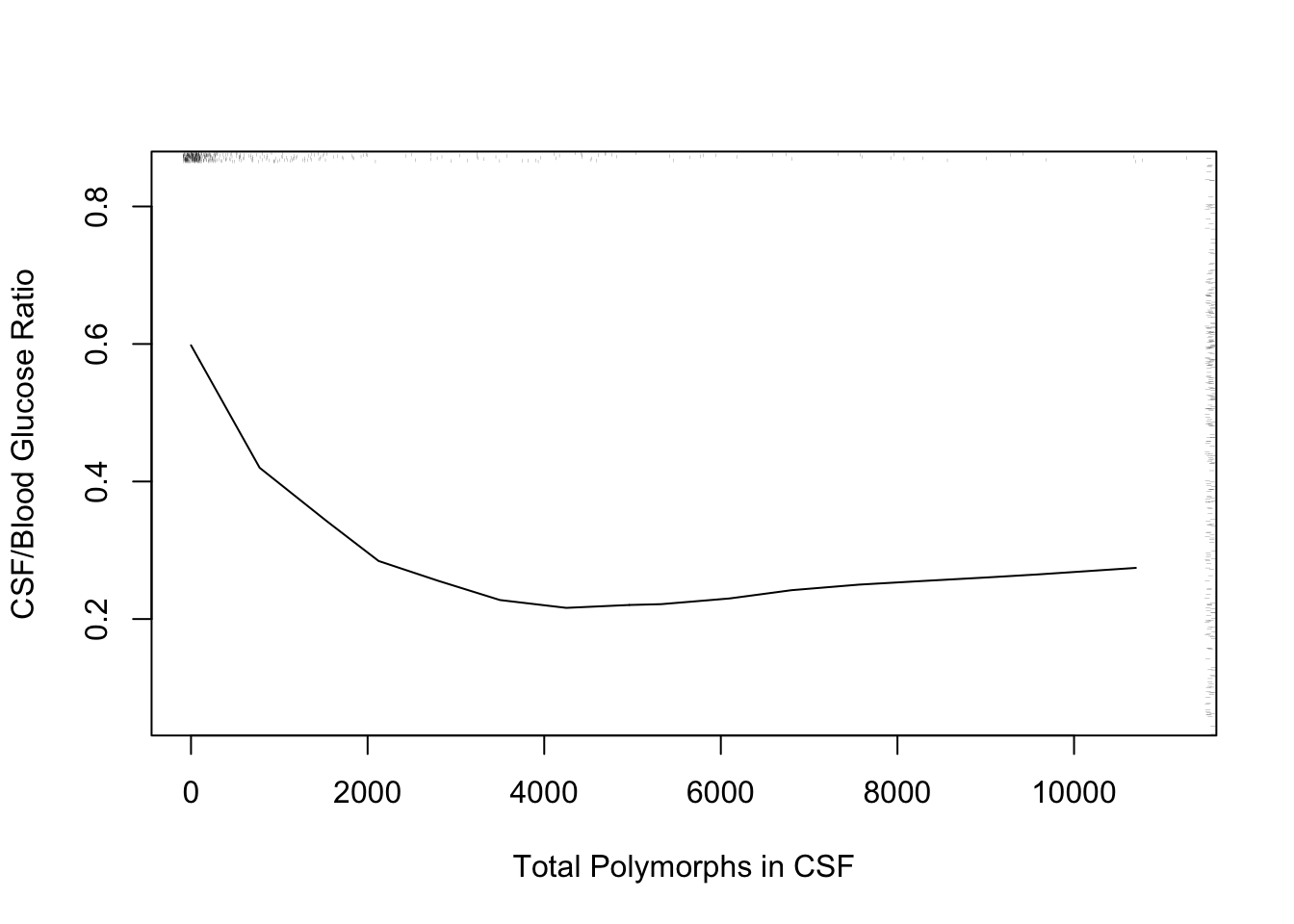Figure 8.6: loess nonparametric smoother relating CSF:blood glucose ratio to total CSF polymorph count in patients with either bacterial or viral meningitis. Rug plot on axes plots indicate raw data values.

The following example using “super smoother” of Friedman (1984) in place of loess to estimate a complex relationship that has a sharp turn.

Code
r <- ABM[, {
plsmo(age, abm, 'supsmu', bass=7,
ylab='Proportion Bacterial Meningitis')
scat1d(age) } ]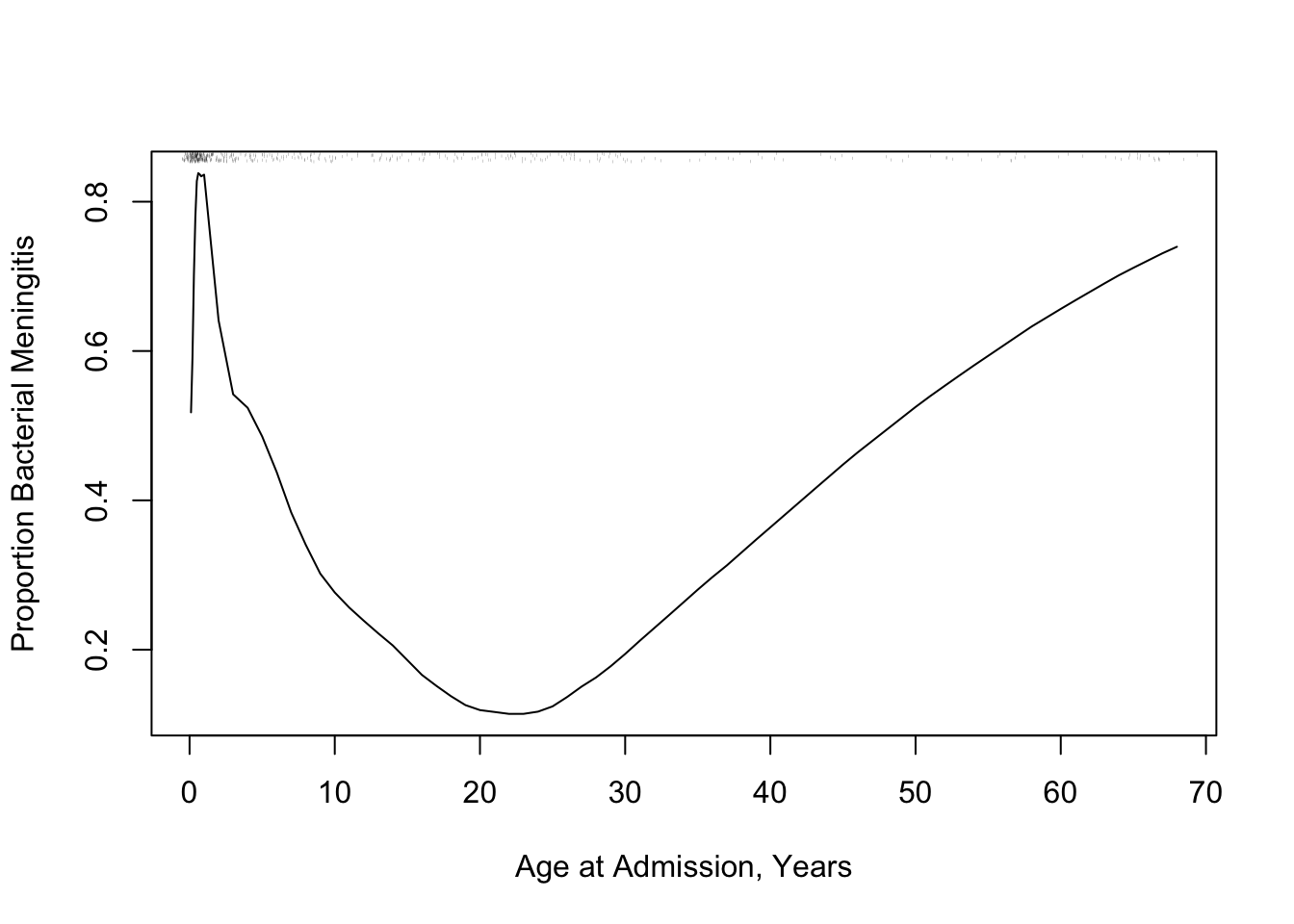Figure 8.7: Super smoother relating age to the probability of bacterial meningitis given a patient has bacterial or viral meningitis, with a rug plot showing the age distribution.
• In both examples there is strong non-linearity, even non-monotonicity
• But loess and the super smoother are limited to estimating a mean or proportion
• Idea of moving average can be extended to all types of $$Y$$, even censored survival times
• Consider overlapping windows in which we compute moving statistics in general
• See here for details and several examples
• Brief idea:
• create overlapping moving windows of $$X$$
• for each window compute the statistic on $$Y$$
• link it with the mean of $$X$$ for that window
• advance $$X$$ by a small amount and repeat
• Smaller windows $$\rightarrow$$ noisier estimates of $$Y$$ but avoid bias
• Smooth the moving estimates using loess or super smoother when finished
• To handle skewed $$X$$ it’s best to form windows on the basis of sample size rather than on absolute $$X$$
• General R function movStats in Github, accessed with the Hmisc package getRs function
• Default algorithm:
• sort the data by $$X$$
• get a target grid of $$X$$ values for which to estimate $$Y$$ separately
• take 15 observations to the left of the target $$X$$ and 15 to the right as the window
• for each new calculation move up $$\frac{n}{200}$$ observations (1 observation if $$n < 200$$) to form the new window
• Example using the glucose data but estimating 3 quartiles plus the mean
Code
getRs('movStats.r')
u <- movStats(glratio ~ tpolys, data=ABM, pr='margin')
Window Sample Sizes
N Mean Min Max xinc
283 30.8 25 31 1
Code
ggplot(u, aes(x=tpolys, y=Moving Median)) + geom_line() +
geom_ribbon(aes(ymin=Moving Q1, ymax=Moving Q3), alpha=0.2) +
geom_line(aes(x=tpolys, y=Moving Mean, col=I('blue'))) +
xlab('Total Polymorphs in CSF') +
ylab('CSF/Blood Glucose Ratio') + ylim(0, 1)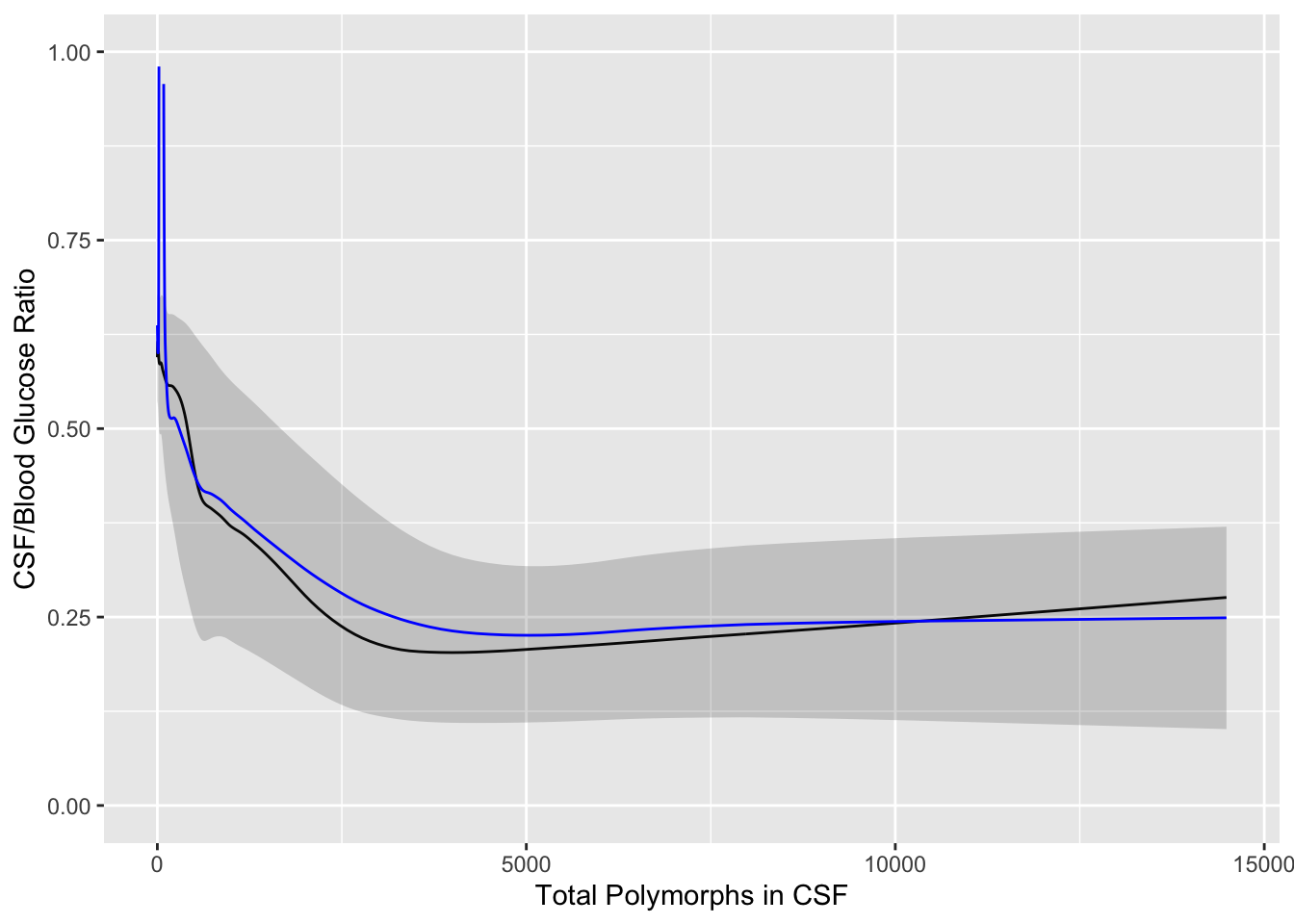Figure 8.8: Moving quartiles and mean (blue line) to relate CSF:blood glucose ratio to total CSF polymorph count in patients with either bacterial or viral meningitis. Black line is the moving median. The band depicts outer quartiles.
• Age example
Code
getRs('reptools.r')   # provides addggLayers
u <- movStats(abm ~ age, msmooth='both', data=ABM, melt=TRUE, pr='margin')
Window Sample Sizes
N Mean Min Max xinc
420 30.9 25 31 2
Code
g <- ggplot(u, aes(x=age, y=abm)) + geom_line(aes(col=Type)) +
ylab('Proportion Bacterial Meningitis')
g <- addggLayers(g, ABM, value='age', type='spike')
addggLayers(g, ABM, value='age', type='ebp', pos='top')Figure 8.9: Moving proportions relating age to the probability of bacterial meningitis given a patient has bacterial or viral meningitis, with a spike histogram and an extended box plot showing the age distribution. Moving proportions before smoothing are also shown. To the right of the extended box plot is a dot for the 0.99 quantile of age
• We also need to estimate the relationship between continuous variables and time-to-event when follow-up is incomplete
• Example: VA lung cancer dataset
• For each moving window compute the Kaplan-Meier 6m and 12m survival estimates
• Subtract cumulative survival from 1.0 to get cumulative incidence
Code
getHdata(valung)
u <- movStats(Surv(t / (365.25 / 12), dead) ~ age, times=c(6, 12),
eps=25, data=valung, melt=TRUE, pr='margin', tunits='month')
Window Sample Sizes
N Mean Min Max xinc
137 48.7 35 51 1
Code
g <- ggplot(u, aes(x=age, y=incidence)) + geom_line(aes(col=Statistic)) +
guides(color=guide_legend(title='')) + ylab('Mortality')
addggLayers(g, valung, value='age', type='spike')Figure 8.10: Moving 6m and 12m mortality as a function of age using VA lung cancer dataset